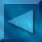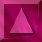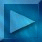Previous : How to choose...  Next : Calculus of the Likelihood Notations

 Choice of the estimator

How to automatically select the optimal hyperparameters?

 We have to optimize a criterion which depends on the hyperparameters.  It is the Maximum Likelihood Estimator on the observed image Y w.r.t.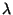and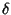: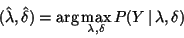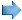maximizing a probability w.r.t.and: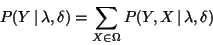where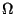= set of images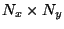, with M grey levels

We use Bayes law to calculate the likelihood :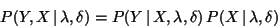which exhibits the two following probability densities :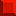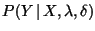probability to obtain the data Y from the initial image X, (joint likelihood of X and hyperparameters)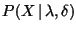a priori probability on the image X  calculated only taking into account the constraints characterizes the type of regularization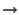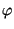-function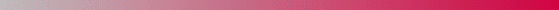André Jalobeanu - 24 Aug 1998